Last year, Jeff Shaffer, the first person to build a sankey in Tableau, posted a blog titled, Sankey Diagrams: Why I Used the Sigmoid Functionand Why You Probably Shouldn’t. In the blog, he discussed the fact that the sigmoid curves used to draw most sankeys in Tableau have a fundamental problem as the curve tends to narrow in the middle preventing it from maintaining an equal width from beginning to end. He suggested that a better approach would be to use sine curves instead of sigmoid curves as the narrowing impact is much less severe. Jeff’s blog provided the following visual to show the issue and compare different curve types (based on the work of Chris DeMartini).But, as Jeff pointed out, this is still not perfect. There is definitely still some narrowing in the middle of the sine curve.

The Problem
There are two things that cause this, the first being the approach used to draw the curve. To demonstrate, let’s start out with two equal-sized bars on either side of our sankey.Now we’ll draw one curve connecting the tops of each bar. Note: I’m using a sigmoid for this example.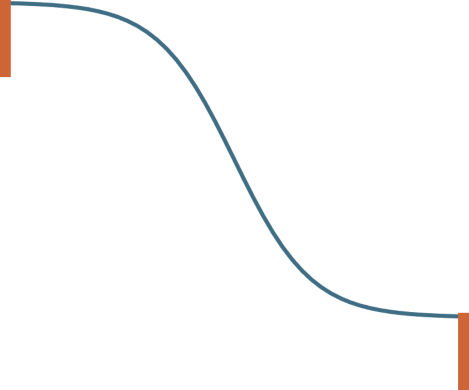Next, we’ll connect the bottoms of each bar using the same kind of curve.We can now clearly see the narrowing in the middle. The approach of drawing two separate curves to connect the top and bottom simply does not guarantee equal spacing between the two curves.

The second problem we encounter has to do with the sizing of the sheet. Take, for example, the followingWe can see the first problem in this example. But what if we resize the sheet after placing it on a dashboard? In the image below, I’ve kept the same height but have made the sheet more narrow. This exacerbates the problem—the start and end of the curve are the same width as the previous image, but the narrowing effect on the middle of the curve is more severe.So, the size of the sheet on a dashboard is also critical when considering a method to draw equal width curves.

Note: While both of these problems are more pronounced with sigmoid curves, as Jeff has pointed out, other curve types have the same issue.

A Potential Solution
A solution to this problem will have to address both of the items above—it must ensure that the distance between the curves remains consistent and it must address the sizing problem. Fortunately, at the end of Jeff’s blog, he included a link to a post by Sam Calisch on Github, from 2011, which describes a mathematical approach to drawing sankey curves which maintain a consistent width. I had come upon this research before and, when I saw it referenced again in Jeff’s blog, it got me thinking. I wondered if I could implement this technique in Tableau in order to address this problem once and for all.

So, let’s talk about Sam’s method a bit. His post includes the following image which does a pretty good job of summing up his approach.There’s a lot going on here, so let me try to break this down into a pieces. There are essentially three components of Sam’s curves: a set of concentric circles on both the left and right and a rectangle in the middle. The distance between the inner and outer circle is the same as the width of the rectangle. These shapes are then connected as shown below.The excess parts of the circle are then removed, leaving partial concentric circles on each end.This is a very clever solution because it is easy to ensure that the width remains consistent when using rectangles and concentric circles.

To create these, we need to know a few key things:

t: The width of the flow
θ: The angle from the top point of each circle to the end point where it meets the rectangle.
r: The radius of the inner circles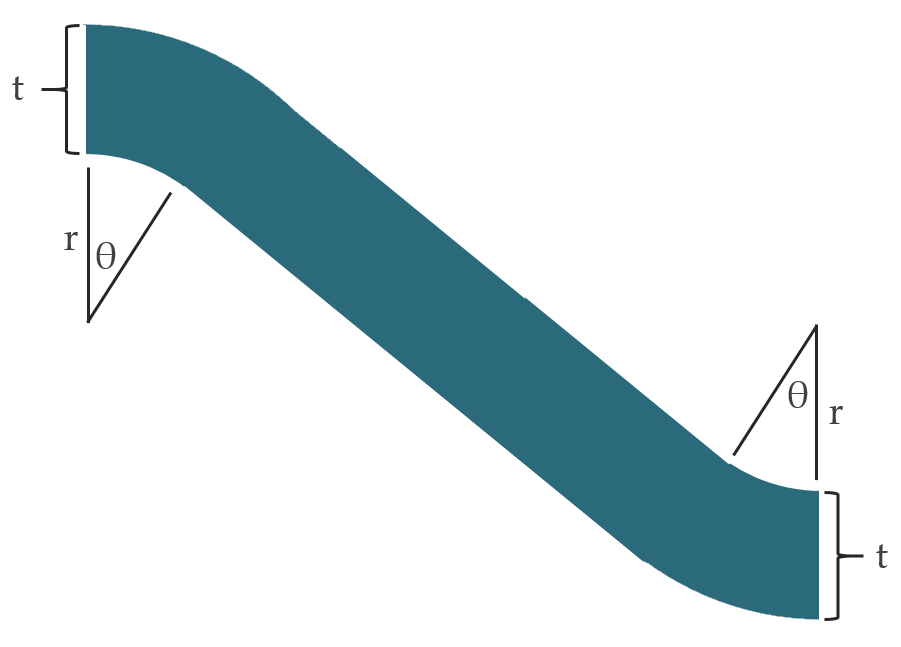With these known, we can use trigonometry and geometry to calculate and plot the semi-circles and the connecting rectangle. Fortunately, t is easy to come by because it’s the width of the flow, which will be determined by some measure in our data set. But calculating r and θ are not nearly as straightforward. Here is Sam’s explanation:

In the following, I’ll describe my method for computing the curves. It could be possible to use splines, but care must be taken to ensure the flow has constant thickness at all points. In order to do this, I use a region defined by concentric circular sections, followed by a sloped rectangle, followed by the same concentric circular section region (but rotated 180°)…

Using the conventions shown in these figures, the variables must satisfy

We set r = ¼(x2 - x1) (for no other reason than that it seems to work) and solve for θ. Without loss of generality, say (x1, y2) = (0, 0). Thus, θ is determined by (x2 - x1), (y2 - y1), and t. Note that if θ0 solves the system for a choice of (x2 - x1), (y2 - y1), and t, then it also solves the system for a(x2 - x1), a(y2 - y1), and at for a scale factor a. In loose language, the same θ works for a short, thin strand as well as a long, thick strand of the same slope. This means we can eliminate one variable. If we precompute a reasonable grid over the resulting two-dimensional space, we can avoid doing any equation solving...I’ll give you this look-up table.

I have to admit that this math kind of blew my mind. I could not understand how to solve this equation in any other way than by brute force experimentation. And, unfortunately, I could not find the lookup table he referenced.

Our Solution
That’s when I decided to call in some help. I sent a message to my brother, Kevin, asking if he had any idea how to solve this equation for r and θ. He was on vacation at the time so I figured he wouldn’t have any time to even think about it. But, after a few back and forth questions, he came back with an absolutely brilliant suggestion. Instead of using the method described by Sam Calisch, what if we did something completely different? He sent me the following that he drew on his phone while lounging on the beach: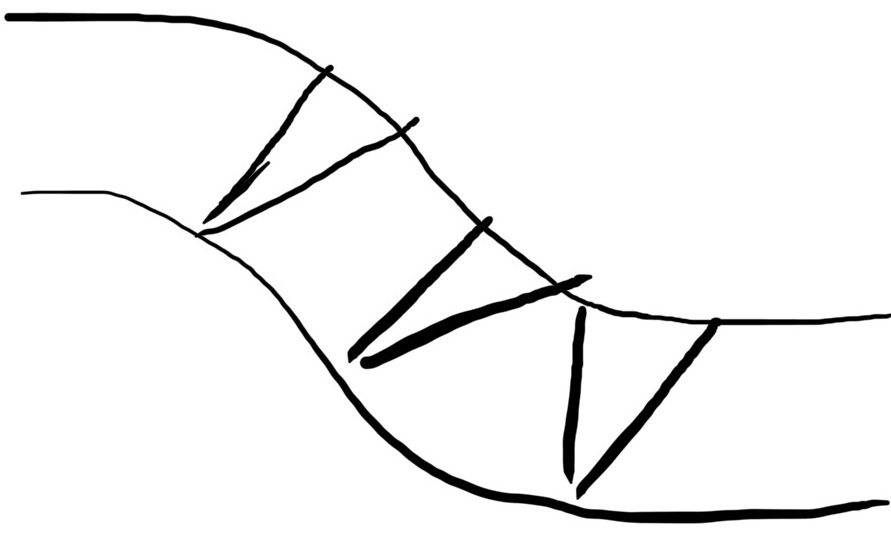His idea was to start by drawing one connecting curve on the bottom. Because curves drawn in Tableau are really just a series of straight lines connecting dots that are very close together—so close that you cannot detect it—we could use some algebra and geometry to first find a perpendicular line, then follow that perpendicular line up until we until we reach the target width of the curve. Then we plot a point there. We continue this process for each point on our curve and, once complete, we’ll have a series of points that are exactly the same distance from the first curve. Finally, we connect those points to draw our top curve.

This was absolute genius!!! (Thanks Kev!!) Had he not came up with this solution, I fear I’d still be beating my head off of the wall trying to solve Sam’s equation. But, seeing Kevin’s solution, I knew it would work. I then fleshed out his idea a bit further on a piece of paper:Let me expound a bit on my explanation above. Here’s the basic process we’ll take:

1) Plot the points along the bottom curve.

2) Calculate the slope of each point. A point doesn’t really have a slope (only lines do), so we’ll actually calculate the slope of the two lines connected to the point, then average them together. This averaging might cause some slight variances from an actual slope, but there are so many individual line segments that these variances will not be of any relevance.

3) Based on the slope, we’ll find the slope of a perpendicular line.

4) Convert the slope of the perpendicular line to an angle.

5) Use the angle and the radius (the width) to find the opposite point (using trigonometry).

6) Repeat this process for each point, then connect those opposite points to create the top curve.

Building it in Tableau
Note: I’m a Tableau junkie and my ultimate goal was to solve this problem and templatize it so that people can create equal-width sankeys in Tableau (I’ll get to that shortly), but I want to note here that the solution documented above could be implemented in any tool or programming language used to create data visualizations. And we believe that it has some potential benefits over some of the existing methods for drawing equal-width curves, which we’ll get to shortly.

I started out by just trying to connect two sets of dots in Tableau. I won’t be going into the calculations in detail, but I essentially implemented the steps detailed in the previous section (if you’d like to see the calcs, feel free to download the workbook—I’ve added comments to make them as easy to understand as possible). After fighting with the calcs for a while, I finally produced this:I have to admit that I was pretty excited about this and immediately shared it with Kevin—his solution had worked!! But, after looking at it for a bit, I noticed a problem. The curve on the top right takes a wider turn than the one on the bottom left. The problem was that I was drawing the bottom curve, then using the math to draw the top curve. Because there is less space on the left/inside of the bottom portion, it squishes the curve on that end.

To correct this, we need to draw a curve in the middle instead of the bottom, then use the perpendicular line approach to extend the curve to the left/top and right/bottom. By drawing the curve in the middle, we could guarantee a uniform curve throughout.

I reworked my calculations and created this:The difference is subtle, but this method does produce a much more uniform curve than my previous attempt.

Integrating it into a Sankey
With the concept proven, I needed to integrate this method into a sankey. While I was able to leverage a lot of the setup from previous sankeys, the new curve method required a complete overhaul of the calculations. But, in the end, I was able to make it work. Here’s a simple animation showing the differences between the old method and the new method.While the difference is somewhat subtle, it is very noticeable in some of the curves in the middle.

And what’s great about this method is that the math will work for any type of curve and will always guarantee the same width across the entire flow. To demonstrate, here’s the same sankey using a sine curve (instead of the sigmoid curve used above):Resizing Problems
So that solves the first problem—the flaws in the curve drawing approach. But what about our second problem? As discussed earlier, if we resize a sheet on a dashboard, it will cause distortions. Unfortunately, this new method suffers from this problem as well. Here’s an example of the new method with a narrowed worksheet:While we do see some distortion, it’s not particularly severe—it doesn’t impact this method quite as much as the previous method. But, since our goal is to ensure consistent width along the entire curve, we’ll want to address the problem. So what’s causing this flaw? When we change the dimensions of the sheet, the width of one unit (along the x axis) is no longer the same as the height of one unit (along the y axis). So, when the sheet is thinner than it is tall, it causes the curve to become thinner the closer it gets to vertical. The opposite is true when you make the sheet wider than tall—the curve gets thicker the closer you get to vertical. To correct this issue, we’ll introduce a parameter that allows us to artificially force the height and width of one unit to be equal. I’ll explain this a bit further in a moment.

To ensure that we always have an equal-width curve, we really need the sheet to always be a perfect square—so that the width and height of one unit is always equal. That, of course, is rarely an option for most data visualization developers, so we’ll need some technique for making adjustments. Unfortunately, this cannot be automated in Tableau as there is no way to get the dimensions of a sheet, as it is configured on a dashboard, within calculated fields. However, there is a relatively simple solution to the problem. To address this flaw, we’ll need to set a different maximum x coordinate based on the amount of “squish” we apply to the sheet. By doing this, we’ll ensure that a unit always has the same width and height.

Let me give you an example to help explain. By default, each set of sankey curves are plotted on a 1 x 1 square grid.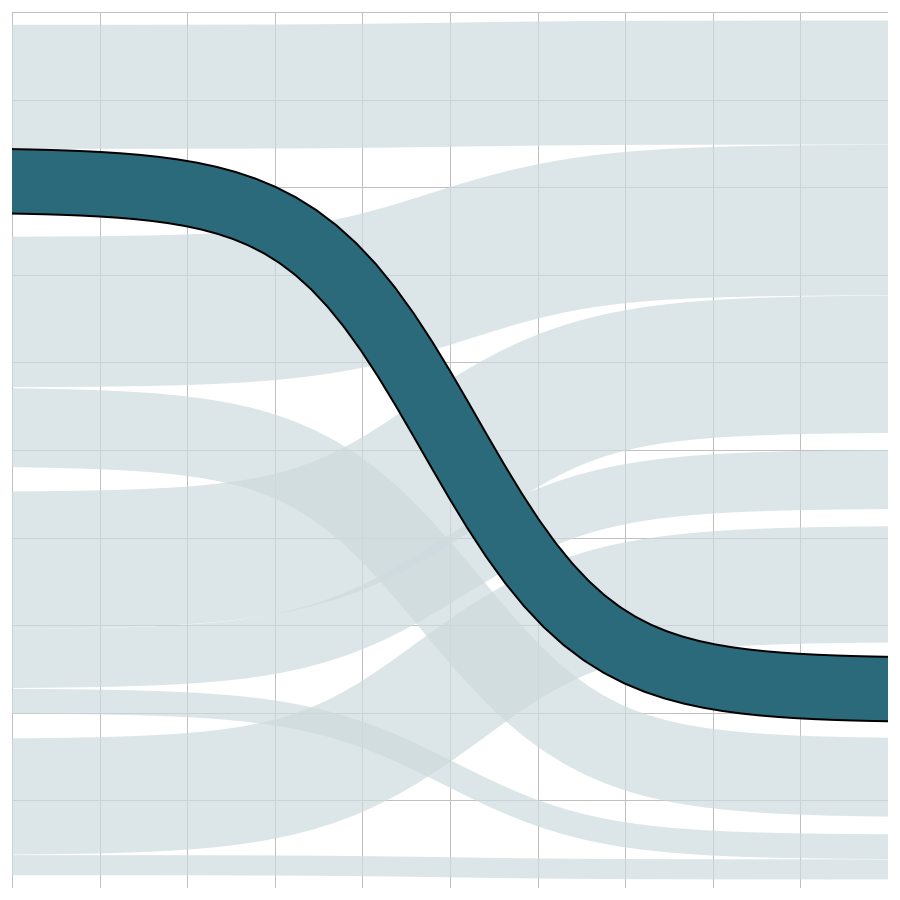The above sheet is 900 px wide and 900 px tall. But, if we adjust the width to 300 px, we can see the narrowing of the grid, which causes the narrowing of the curve.Each unit of the grid now has a width that is 1/3 (0.33333…) of its height. But if we adjust this so that the curve is drawn from an x coordinate of 0 to 0.33333, each unit of our grid will return to equal width and height—we’re just not drawing as far to the right. If we then fix the x axis to 0.33333, we’ll have something like this.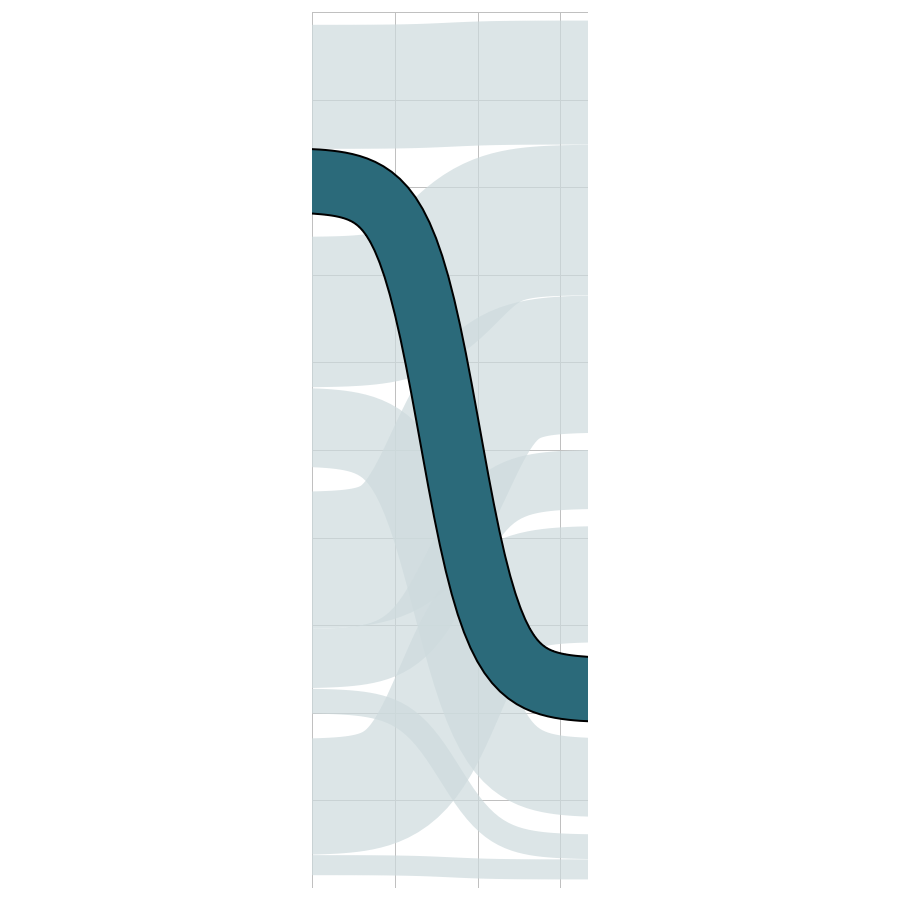Notice that each square in the grid how has the same width and height. And, as you can see, the curve is now a consistent width throughout. So, with this ability to make these fine-tuning adjustments, we are now able to solve our second flaw as well.

The Template
With both of these problems addressed, we are now able to create sankey curves that are guaranteed to have a consistent width!! So, my final step is to templatize this approach so you can easily plug in your own data and produce an equal-width sankey for yourself. I’ve previously created templates for regular sankeys, multi-level sankeys, traceable sankeys, gradient sankeys, and sankey funnels. I won’t be providing separate templates for all of these. Rather, I’m going to provide a single template for a multi-level sankey. This template will automatically work as a sankey funnel (if you have nulls in any of your steps) and can be easily modified to work as a single-level sankey. Gradient sankeys aren’t terribly practical, so I’m leaving those out. I’m also excluding the traceable sankeys for now, but, if there is enough demand, I may consider creating traceable versions in the future.

Like my other templates, this one includes two components—an Excel spreadsheet and a Tableau workbook. The Excel spreadsheet has two sheets, Data and Model. Model is used to handle the data densification needed to draw the curves (Note: This model is different than previous templates). You don’t need to worry too much about this sheet—just make sure it’s in your spreadsheet. Data is used to populate your data. It contains columns for each of the steps, plus a Size field for the measure you’ll be visualizing.

Next download the Tableau template. Then edit the data source and connect it to your Excel file. The workbook should update automatically to reflect your data.

The workbook comes with three different curve types—Sigmoid, Sine, and Cubic (thanks to Chris DeMartini for his work on different curve types). By default, the curve is set to use Sine, but you can change it using the Curve Type parameter.

Like previous templates, the workbook also allows you to make the whitespace configurable. You can change this using the Whitespace parameter.

To adjust your curves to account for the size of the sheet on the dashboard, you’ll need to do the following:

1) Calculate the ratio of width to height. To do this, click on the curve sheet, go to the Layout panel, find the width and height, then divide width by height.
2) Enter this value into the Squish Ratio parameter.
3) Edit the x axis on each curve sheet, setting the “fixed end” to use this value.

And that’s pretty much it. From here, you can do whatever you like with the chart—change the colors, add filters, update tooltips, etc. just as you normally would.

I’ve placed all the files in the following publicly accessible location. I’ve included the Excel template as well as workbooks in both 2019.4 and 10.4 formats.

Wrap-Up
This was a fun (and very challenging) project. If you’ve read through this whole post, thank you for indulging my extreme verbosity. I wanted to make sure I thoroughly explained all of the issues I was attempting to solve as well as show the various iterations I took to arrive at a solution. I hope you enjoyed this read and use this new sankey approach in your work. If you have any thoughts or comments, please leave them below. Or, if you have questions, need assistance, or experience any problems, feel free to reach out to me.

Ken Flerlage (with help from Kevin Flerlage), January 6, 2020

1.1.